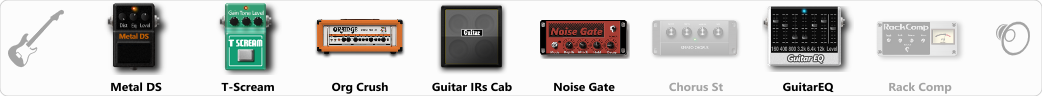# Deathcore/Satan

Discussion in 'ToneLib-GFX presets' started by braian, Jun 10, 2019.

1. Deathcore/Satan

GFX version: 3.0

Preset name: Deathcore/satan
Optimized for: Phones/Speaker

Effects chain:guitarra cort x1, pickups emg 81, interfaz behringer U-PHORIA UM2

Effect: "Metal DS" (Overdrive / Distortion), active - "yes"
"Dist" = 19
"Bass" = 61
"Middle" = 25
"Treble" = 24
"Level" = 46

Effect: "T-Scream" (Overdrive / Distortion), active - "yes"
"Drive" = 22
"Tone" = 80
"Level" = 79

Effect: "Org Crush" (Amp simulators), active - "yes"
"Gain" = 65
"Bass" = 71
"Middle" = 55
"Treble" = 56
"Presence" = 55
"Master" = 72
"Output" = 50
"Level (dB)" = 0

Effect: "Guitar IRs Cab" (Cabinets), active - "yes"
"Model" = Vox AC30 (2x12")
"Mic Position" = Middle
"Mic Distance" = Near
"Low Cut (Hz)" = 60
"Hi Cut (kHz)" = 20.0
"Mix" = 100
"Level (dB)" = 0

Effect: "Noise Gate" (Dynamics / Filter), active - "yes"
"Detect" = Gtr In
"Mode" = Auto
"Depth" = 50
"Threshold" = 50
"Attack" = 0
"Hold" = 5
"Decay" = 785

Effect: "Chorus St" (Modulation / Sfx), active - "no"
"Speed" = 0.1
"Depth" = 32
"Center" = 4.2
"Mix" = 69

Effect: "GuitarEQ" (Dynamics / Filter), active - "yes"
"160 Hz" = 3
"400 Hz" = 0
"800 Hz" = 0
"1.6 kHz" = 5
"3.2 kHz" = 0
"6.4 kHz" = 3
"12 kHz" = 0
"Level (dB)" = 0

Effect: "Rack Comp" (Dynamics / Filter), active - "no"
"Threshold (dB)" = -20
"Ratio" = 5
"Attack" = Slow
"Release (ms)" = 250
"Knee" = 25
"Level (dB)" = 0

Note: This is a ToneLib-GFX preset file, you will need to download and install the ToneLib-GFX software to use the preset.

#### Attached Files:

• ###### Satan.tlgfx
File size:
722 bytes
Views:
5,255
mizvv, Eryukovskiy, loshara and 18 others like this.